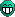## Accumulator / Solar panel ratio

Post all other topics which do not belong to any other category.
Cilya
Inserter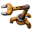Posts: 26
Joined: Mon Mar 24, 2014 4:07 pm
Contact:

### Accumulator / Solar panel ratio

Hello everyone.

I was thinking about the energy model of factory, when i decided to look over the internet what people were actually doing. I found a nice design from Cellidor on reddit. Using blueprint and roboports for solar panel farms is especially nice since it allow you to scale the energy production very fast to match the huge consumption that using productivity modules implies. Otherwise it's a lot more time invested in the building of those, and you spend much more time placing solar panels than doing anything else. Moreover, it allow your solar panel farm to be a safe way for drones. Space is generally not the rarest resource in factorio.

However, I was not happy with the solar panel / accumulator ratio in this design. Without good reason at first, only a disturbing feeling. I decided to resolve the question of the ratio a bit more rationally. This is what i will explain below. Please tell me if i've done any error, i'm very interested in this.

edit: As pointed out by ssilk, this question has already been discussed several times. A list of forum topics discussing about this can be found on the wiki page for solar panels.

How to compute the optimal Accumulator / Solar panel ratio.

Let's say that our factory is using an average power P and that we want to power it only with solar panels and accumulators. Since solar panel are the only outpur of electricity, they need to generate during a whole day enough energy E_sol to provide an average power P during this time. . Let's note t1 the day time, t2 the night time and t3 the dawn or dusk time which are the same and T = t1+t2+2*t3 a whole day time. The energy E_sol is :

E_sol = P * T = P * (t1 + t2 + 2 * t3)

Since the daylight allows them to produce electricity only during a fraction of a day, they need to provide an output power P' greater than P to not only power the factory during the day but also charge the accumulators which will power it during the night. The energy produced during a day by the solar panel is the sum of the power outputed on each game tick and can be computed as the area of the trapezoid described by the solar panel power curve, represented in red below.This trapezoid has height P' and sides t1 and t1+ 2 * t3. Thus we can also define E_sol as

E_sol = P' * (t1 + t1 + 2*t3) / 2 = P' * (t1 + t3)

Using the two previous equations we have

E_sol = P * T = P' * (t1 + t3)

frow what we can compute P' as

P' = P * T / (t1 + t3)

The capacity storage needed for the accumulator to maintain the output power P during dusk, night and dawn (represented as a blue curve above) can also be calculated as the area of the trapezoid below the accumulator power curve. This trapezoid has height P but its sides needs to be calculated. The accumulators starts to output power when the solar panels output falls below P. Since their output power falls linearly from P' to 0 in time t3, the time during which the accumulator output is growing is t3 * P/P'. Thus we have that the energy E_acc restored during the night is

E_acc = P * (t2 + t2 + 2 * (t3 * P/P')) / 2 = P * (t2 + t3*P/P')

Now, say we want to compute then number of accumulators n and the number of solar panels m needed to provide the target power P at any time. They can be computed from the capacity c of one accumulator and the power output o of one solar panel.

n = E_acc / c
m = P' / o

Finally, the ratio of these two values can be computed :

n / m = (E_acc / P') * (o / c) = [(t1 + t3) * [t2 + t3 * (t1 + t3) / T] / T] * (o/c)

We can get the values from the wiki.

o = 60 kW
c = 5000 kJ
t1 = 0.5
t2 = 0.1
t3 = 0.2
T = 1.0

Times are in fraction of day, so they must be multiplied by (25 000 / 60). With these numbers, the numerical application gives us :

n / m = [(0.5 + 0.2) * [0.1 + 0.2*((0.5+0.2) / 1.0] / 1.0] * (25 000 / 60) * (60 / 5000) = 0.84

It does mean that for 100 solar panels, we need 84 accumulators.

• This result only apply when solar energy is the only source of electricity production. Otherwise, other sources of electricity production will have the priority on accumulators. In this case, accumulator can provide a temporary increase in power when needed (for instance for laser turret during an alien attack) and their number is not calculated this way and generally much lower.
• During the night, the energy consumption is generally a bit higher since lights are turned on. However, the power consumption from light is generally too small to change this result significantly.
• In general, we build more solar panel than needed to ensure that at any moment, we can provide all the required power. However, I have assumed in my calculation that we had built the exact number of each structure necessary, not one more, not one less. However, the result is relevant in any case. If you don't use this ratio, and if your needs of energy are growing, one of this two situation will eventually happen : either your ratio is below 1.05 and you wont have enough energy stored for the night, or, your ratio is above and you wont be able to fill your accumulators during the day.
• We consider here an average power P needed. In general, there may be important variations of the power consumption. We can probably safely use the average power for the following reasons. During the day, the output power P' is usually enough (42% higher than P) to fill any power requirement. If it's not enough, accumulators can probably fill the difference. The variations of the consumption above and below P will be compensated by the accumulators which will charger slower or faster. (This is the purpose of accumulatrs) During the night, if there is a consumption pick, accumulators have a much higher maximum output power than what they are outputing on average, thus it's very unlikely there will be power shortage.

Exploitation of the result.

A possible exploitation of this result is to define a building pattern for solar panel and accumulators in which the ratio is preserved. Thus, each time the pattern is built, the ratio stay the same.

Here is an example of pattern. It uses roboport on the center so its repetition (with up to two tiles between each pattern, allowing to move between them) is possible and can be built by construction robots automatically. It as a space efficiency of 96.5% (3.5% of the tiles, used by the roboport and the substations, are not used by solar panel and/or accumulators) and an accumulator/solar panel ratio of 0.84.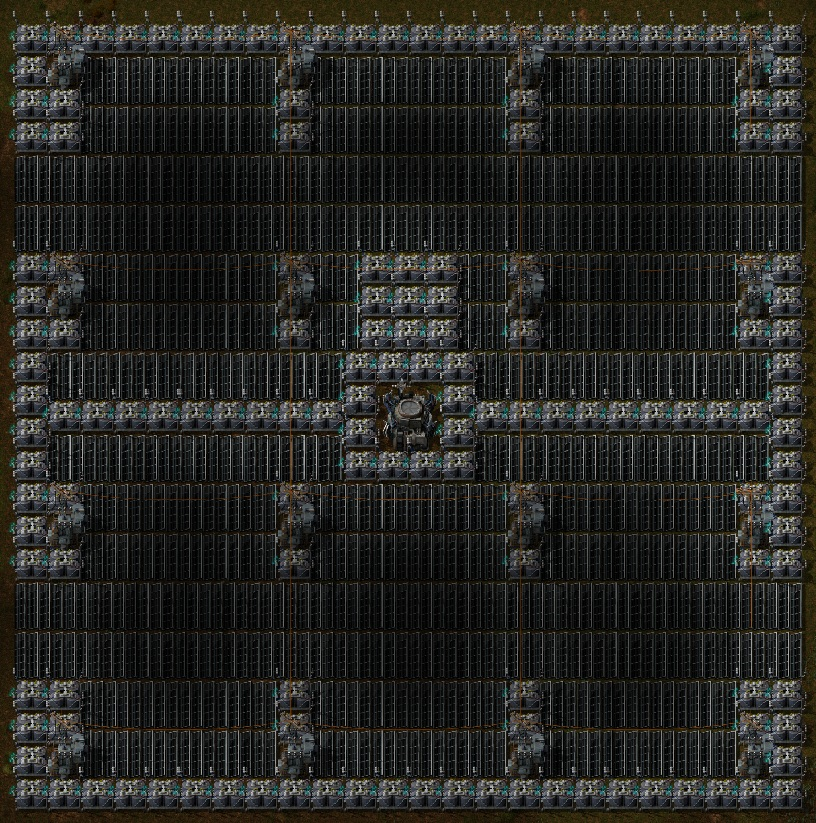Size: 48x48 (2304 tiles)
Usefull area : 2224 (96.5%)
Solar panels: 180
Accumulators: 151
Substations: 16
Roboport: 1
* Logistic network tilable
* Electric network tilable
Last edited by Cilya on Sat Aug 30, 2014 12:43 pm, edited 5 times in total.

ssilk
Global Moderator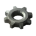Posts: 12686
Joined: Tue Apr 16, 2013 10:35 pm
Contact:

### Re: Accumulator / Solar panel ratio

Cool suggestion: Eatable MOUSE-pointers.
Have you used the Advanced Search today?
Need help, question? FAQ - Wiki - Forum help
I still like small signatures...

Cilya
InserterPosts: 26
Joined: Mon Mar 24, 2014 4:07 pm
Contact:

### Re: Accumulator / Solar panel ratio

Thank you, i didn't found this link. (I was searching in the electric network page mainly, not in the solar panel one) We don't have the same results though. I'll try to dig deeper into their demonstration.

edit: I agree with the second link. Except that the ratio 20:21 is the other way round : an accumulator provides less power during the night (mean 40kw) than a solar panel provides to the factory (mean 42kw) so, you must have more accumulator to balance that. That is 21 accumulator for 20 solar panel. (5%)

DerivePi
Filter Inserter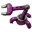Posts: 504
Joined: Thu May 29, 2014 4:51 pm
Contact:

### Re: Accumulator / Solar panel ratio

Let P = 42 kW average power consumption throughout the day
E_night = 42 x (41.7 + 83.3) = 5,250 kJ
E_Day = 42 x (208.3+83.3) + 5,250 = 17,500 kJ
P' = 17,500 / 291.67 sec = 60 kW = 42 x (416.7 / (208.3 + 83.3) ) = 60 kW
n = 5250 / 5000 = 1.05 accumulators
m = 60 / 60 = 1 solar panel

Verified. Well done!

DaveMcW
Smart Inserter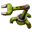Posts: 3604
Joined: Tue May 13, 2014 11:06 am
Contact:

### Re: Accumulator / Solar panel ratio

This post has the correct math: https://forums.factorio.com/forum/vie ... 928#p39928

I edited it slightly here because Fraktal worked with megawatts instead of kilowatts.
Khyron wrote:I'd just do the following math:

P = 42kW
night = 41.66
dawn, dusk = 83.33
How long into dusk do solar panels provide 100% or more of P: 83.33 - 83.33 * 0.7 = 25s
(= the point at which accumulators start to provide power)

Duration of part of dusk where accumulators provide some power, ramping up to 100% of P: 83.33 - 25 = 58.33s
Divide by two since they linearly ramp from zero to 1
Multiply by two since they also do this at dawn.
=58.33 * 2 / 2

So accumulators need to provide P for 41.66+58.33 = 100s

... Interestingly if you have more solar panels than this ratio requires you actually require fewer accumulators since they will kick in later during dusk and stop providing power earlier in dawn.
Now that we know we need 100 seconds of accumulator power, we can plug in the numbers.

42 kW * 100s / 5 MJ = 0.84 accumulators per solar panel.

DerivePi
Filter InserterPosts: 504
Joined: Thu May 29, 2014 4:51 pm
Contact:

### Re: Accumulator / Solar panel ratio

yup, my fault. The energy calculated for night time is incorrect (it excludes the energy where the power exceeds the average power usage towards the day times) and some of that energy is attributed to solar panels during the twilights.

Cilya
InserterPosts: 26
Joined: Mon Mar 24, 2014 4:07 pm
Contact:

### Re: Accumulator / Solar panel ratio

I've fixed it and precised the proof. I believe it's correct now. Thank you !

electriccat
Manual Inserter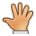Posts: 1
Joined: Wed Sep 24, 2014 7:27 pm
Contact:

### Re: Accumulator / Solar panel ratio

Hi all, great thread on this. Don't know if i'm missing something on this but in the overnight period i'm never seeing a power output occur from the accumulators using this pattern. I've even tried during different daylight periods to have specific grids of just accumulators and substations. I can see that there is excess solar power capacity available but still not seeing an output. Guessing i'm missing something simple. Ideas?

Boogieman14
Filter InserterPosts: 769
Joined: Sun Sep 07, 2014 12:59 pm
Contact:

### Re: Accumulator / Solar panel ratio

Do you still have steam engines in your network? Those take priority over accumulators. If they can cover the demand, your accumulators won't be used at all. There should be a few posts around here about a setup to allow using those steam engines as emergency power only.
I don't have OCD, I have CDO. It's the same, but with the letters in the correct order.

MaxStrategy
InserterPosts: 49
Joined: Wed Feb 19, 2014 11:20 am
Contact:

### Re: Accumulator / Solar panel ratio

Amazing proof Cilya!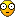sinsiliux
InserterPosts: 32
Joined: Mon May 19, 2014 12:40 am
Contact:

### Re: Accumulator / Solar panel ratio

Among several posts trying to find correct ratios, this one is actually the only one I saw that is correct. I've actually made one myself a while ago, however I made several mistakes. Good job!

Just so everything is in one post:
As you've shown solar panel is active for t1 + t3 = 0.5 + 0.2 = 0.7 of the day.
Which means that solar panel is effective 70% of the time, or you could say that solar panel produces 42KW of power on average.

Given that here's a table to easily find out how many solar panels and accumulators you need to reach desired power output:

ssilk
Global ModeratorPosts: 12686
Joined: Tue Apr 16, 2013 10:35 pm
Contact:

### Re: Accumulator / Solar panel ratio

Cool suggestion: Eatable MOUSE-pointers.
Have you used the Advanced Search today?
Need help, question? FAQ - Wiki - Forum help
I still like small signatures...

Lawvill
Manual InserterPosts: 1
Joined: Wed Dec 31, 2014 11:53 am
Contact:

### Re: Accumulator / Solar panel ratio

I was looking at the page on the day/night cycle, and it suggests that the numbers should be as follows:

Type - Game Ticks - Ratio of a day
Full day - 24966 - 1
Day - 12482 - 0.5
Night - 3994 - 0.16
Dawn or Dusk - 4245 - 0.17

When I put these calculations in the formula, I got a ratio of 0.92, or 23 accumulators to 25 panels.
Have I gone wrong with something? I have only started to play this game a few days ago, and so am not familiar with what changes may have occurred recently.

Source: https://forums.factorio.com/wiki/inde ... e=Game-day

mikehendi
InserterPosts: 32
Joined: Sun Dec 21, 2014 4:41 pm
Contact:

### Re: Accumulator / Solar panel ratio

Without checking your math, the day/night cycle changed a bit in 0.11.8, so that's probably why you now get different results.

jonesiblitz
Manual InserterPosts: 2
Joined: Sat Jan 03, 2015 6:39 pm
Contact:

### Re: Accumulator / Solar panel ratio

I was interested in how the equation simplified down to what is shown, thought I might share the results.

E_acc = P ( t2 + (t3P/P’))
P’=PT/(t1+t3)

E_acc = P ( t2 + (t3P/(PT/(t1+t3))))
= P ( t2 + (t3P/1) ((t1+t3)/PT))
= P ( t2 + (t3/1)((t1+t3)/T))

n / m = E_acc / p’ * o / c

= [P ( t2 + (t3/1) (( t1+ t3)/T))] / [PT / (t1+t3)] * o/c
= [P (t2 + (t3/1) ((t1 + t3) / T )] * [(t1 + t3) / PT] * o/c
= [(t2 + t3((t1 + t3)/T)] * [(t1 + t3) / T] * o/c

t1 = .5
t2 = .1
t3 = .2
T = 1
o = 60
c= 5000

[(.1+.2((.5 + .2)/1)] * [(.5 + .2) / 1] * 60 / 5000 * 25000 /60
[(.1 + .2(.7)] * [.7] * 5
[.1 +.14] * .7 * 5
.24 * .7 * 5 = .84
1/.84 (roughly) 1.19047619047619

Noticed a post with new numbers and a result that once tested is correct. The ticks listed at 24966 I saw somewhere on reddit as well, a person had created a mod that counted ticks to help prove their solar panel ratio. (if someone can find the link to that thread it would be helpful, been looking for a couple days) Here is my calculations with the numbers posted.

New Numbers
Type - Game Ticks - Ratio of a day
Full day - 24966 - 1
Day - 12482 - 0.5
Night - 3994 - 0.16
Dawn or Dusk - 4245 - 0.17
[(.16+.17((.5 + .17)/1)] * [(.5 + .17) / 1] * 60 / 5000 * 24966 /60
[(.16 + .17 (.67)] * [.67] * 24966/5000
[((16/100)+(17/100)(67/100)] * [67/100] * [24966 / 5000]
[((16/100)+(1139/10000)] * [67/100] * [24966 / 5000]
[(1600/10000) + (1139/10000)] * [6700/10000] * [24966/5000]
[2739/10000] * [6700/10000] * [24966/5000]
[18351300/100000000] * [ ( 24966 / 5000) * (20000 / 20000)] (bringing everything to similar denominator)
[18351300/100000000] * [ 499320000 / 100000000] (dropping 100 off all numbers)
[183513 / 1000000 ] * [ 4993200 / 1000000]
916,317,111,600 / 1,000,000,000,000
9,163,171,116 / 10,000,000,000
.9163171116
since you need more you can not round down so roughly .92 or 92 accumulators per 100 solar panels (23:25 or 46:50)
or inversely you get roughly 1.091325249022011 solar panels per accumulator

This change means in the long run you need less solar per accumulator.
Without detailed calculations I believe that solar panels cost more than accumulators. So this change is for the better.

If you see any flaws in my calculations let me know. Thanks

My next task will be to create a new solar field blueprint that is optimized with this data. (Don’t hold your breath though)

DaveMcW
Smart InserterPosts: 3604
Joined: Tue May 13, 2014 11:06 am
Contact:

### Re: Accumulator / Solar panel ratio

0.84 is still correct.
Tested with 50 solar panels, 42 accumulators, and 7 radars in 0.11.8.

It looks like the wiki article was vandalized. game.darkness which the mod measures caps out at 0.85, but solar panels continue to decrease to full darkness a few hundred ticks later.

jonesiblitz
Manual InserterPosts: 2
Joined: Sat Jan 03, 2015 6:39 pm
Contact:

### Re: Accumulator / Solar panel ratio

DaveMcW wrote: game.darkness which the mod measures caps out at 0.85
Can you site where you gathered this information?

DaveMcW
Smart InserterPosts: 3604
Joined: Tue May 13, 2014 11:06 am
Contact:

### Re: Accumulator / Solar panel ratio

/c game.player.print(game.daytime .. " " .. game.darkness)

shinyarceus4
Burner Inserter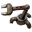Posts: 5
Joined: Sat Jan 03, 2015 1:33 am
Contact:

### Re: Accumulator / Solar panel ratio

I think I'll stick with 8:7 solar/accumulators, thank you very much...

Still, it's interesting to see the exact ratio for optimal power.

Ojelle
Fast Inserter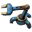Posts: 143
Joined: Thu Feb 12, 2015 9:21 am
Contact:

### Re: Accumulator / Solar panel ratio

For some reason this is my bookmark. Has anything changed yet to the ratio actually? Or not? And is the word 'actually' on its place in that sentence? Its a literal translation from Dutch...
Choumiko wrote:
sillyfly wrote:kovarex just posted the thread... but with #118 in the title. I think they had too much beer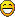It's a wonder how good the game is, if you consider how bad they are with the FFF numbers